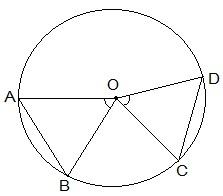Guru

# Prove that if chords of congruent circles subtend equal angles at their centres, then the chords are equal. Q.2

• 0

Give me the best and simple way for solving the question of class 9th of Circles chapter of math of question no.2 how i solve this question in easy and simple way because it is very important type of question of exercise 10.2 Prove that if chords of congruent circles subtend equal angles at their centres, then the chords are equal.

Share

1. Consider the following diagram-Here, it is given that AOB = COD i.e. they are equal angles.

Now, we will have to prove that the line segments AB and CD are equal i.e. AB = CD.

Proof:

In triangles AOB and COD,

AOB = COD (as given in the question)

OA = OC and OB = OD (these are the radii of the circle)

So, by SAS congruency, ΔAOB ΔCOD.

∴ By the rule of CPCT, we have

AB = CD. (Hence proved).

• 0